# Programming - Java Graph Coloring Algorithms (Backtracking and Greedy)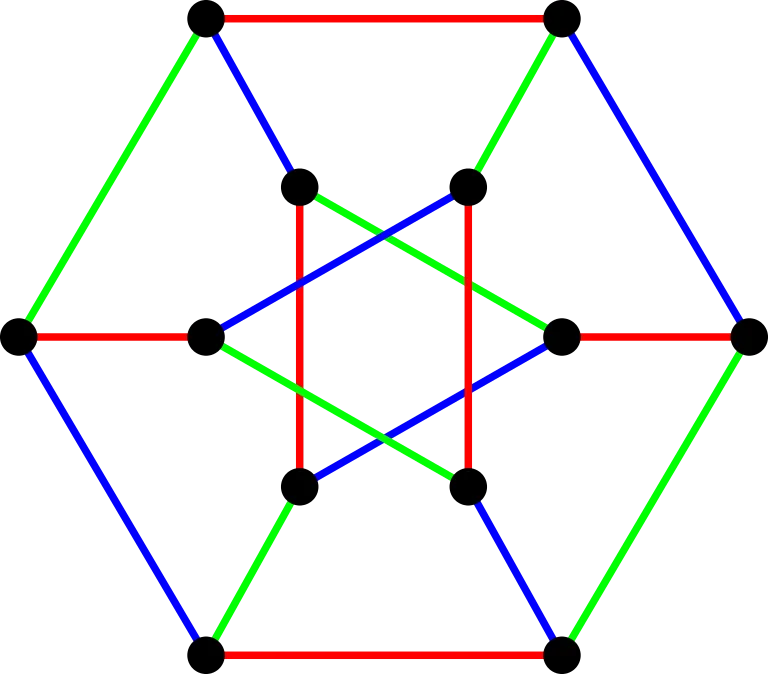[Image source:]

All the Code that will be mentioned in this article can be found at the Github repository:

https://github.com/drifter1/javagraphalgorithms

## Introduction

Hey it's a me again @drifter1! Today we continue on with the Java Graph Algorithm series to cover Graph Coloring Algorithms. The two algorithms that I want to cover are the Greedy (simple algorithm) and Backtracking ones. As always we will first start with some Theory about the topic of Graph Coloring, and then get into the actual implementation of those algorithms in Java, using knowledge of the previous articles/codes.

So, without further ado, let's get started!

## Graph Coloring (in general)

Graph Coloring is the problem of assigning colors to certain elements of a graph, by also following certain constraints. The most common graph coloring problem is Vertex Coloring, which is what we will cover today. In this problem we are given m colors that we have to "apply" to the vertices, in such a way that two adjacent vertices do not have the same color. Other problems are Edge Coloring, where two incident edges can't have the same color and Face Coloring, which can be transformed into Vertex Coloring.

For a graph we define it's chromatic number as:

The smallest number of colors needed to color a graph G.

[Reference_1]

Calculating/Finding the chromatic number of a graph is a NP Complete problem, which means that it's of Polynomial time complexity and can be solved by a non-deterministic method/algorithm.

Graph coloring is used in (Applications):

• Time Tables or Schedules
• Sudoku, which is a variation of the Graph coloring problem
• Register Allocation in Compiler Optimization
• To check if a graph is Bipartite
• Geographical Map Coloring
• and even more...

Let's get into Algorithms that solve this problem...

## The simple "Greedy" (or dump) Algorithm

The simplest and most basic algorithm that can solve the Problem of Vertex Coloring is of course the Greedy Algorithm. Such an algorithm doesn't use the minimum number of colors, but just guarantees an upper bound of maximum colors. The algorithm just can't use more then d+1 colors, where d is the maximum degree of a vertex. This shows us that this algorithm at worst gives us a Coloring that uses (d+1)-colors.

The Pseudocode/Procedure of this Algorithm goes as following:

1. Color the first vertex with the first color

2. For the remaining vertices:

a) Color the currently picked vertex with the lowest numbered color that has not been used in adjacent vertices to it (neighbours).

b) When all previously used colors appear on adjacent vertices, then we assign a new color to it.

3. Print the resulting color assignments

### Implementation in Java

The Implementation of this and the next algorithm in  Java follows the simple "Adjacency List"Graph  implementation without Edges, that we implemented in the first article of the series.

This means that the Graph class is: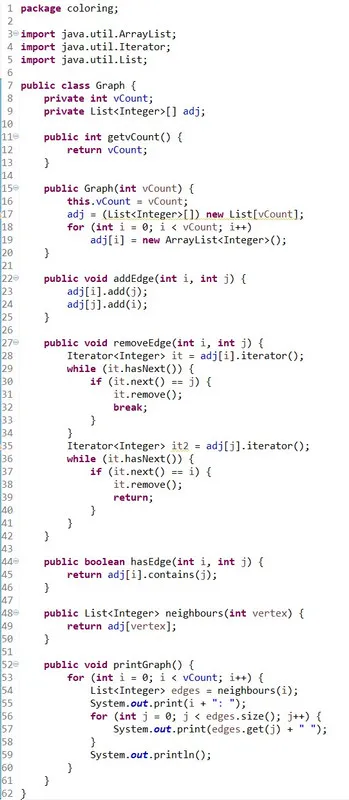[Eclipse Screenshot]

The actual implementation of this algorithm looks as following: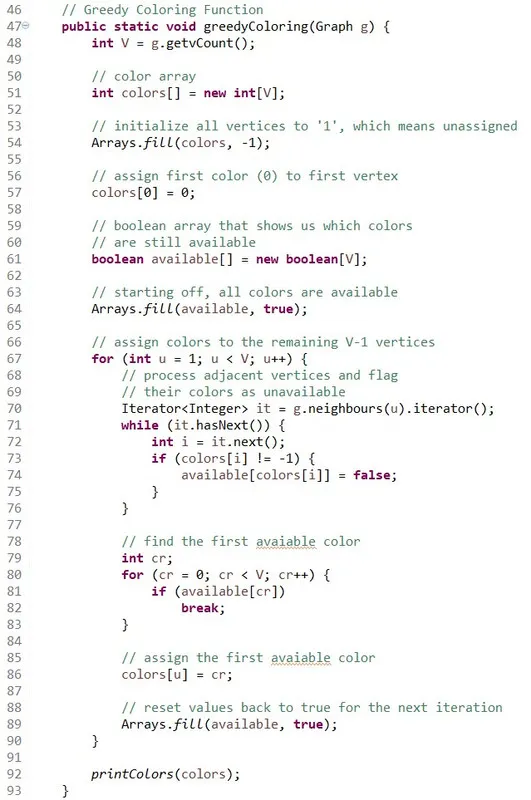[Eclipse Screenshot]

### Code explanation:

You can see that we define two arrays:

• colors[] -> where we store the colors of the vertices
• available[] -> that helps us check which colors are availabe. Checking which colors are already used by neighbours check in other words.

To fill the Arrays we use the Arrays.fill() function, that is pretty handy!

In the Graph object/class I have defined a function that returns the neighbours (adjacent vertices to a vertex) as a linked list of integers. By doing that we can now use a List Iterator called "it" to iterate through the List with ease. Storing the actual End-Index of each Edge, starting of from Start-Index 'u', inside of this Neighbours-List, we just have to get this index 'i' and set the corresponding available[]-index, if this Edge exists.

To print out the colors are defined the following function in TestGraphs: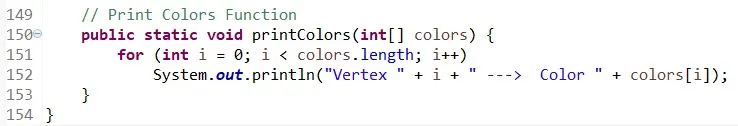The actual result (Console) that we get for a specific Graph, comes after the next algorithm implementation...

## The more advanced "Backtracking" Algorithm

Given an undirected Graph we can also determine if this Graph can be colored with at most m colors, using a Backtracking Algorithm, that tests out different Cases.

The approach of this algorihtm can be summarized as:

while there are untried configurations
{
generate the next configuration
if no adjacent vertices are colored with same color
{
print this configuration;
}
}

[From Reference_3]

The idea is to assign the colors one by one to different vertices, starting from vertex 0. Before assigning this color, we first check if it is "safe" to assign it, by considering the already assigned colors of the neighbours. When safe then the color assignment becomes a part of the solution. If we don't find a color due to clashes, then we backtrack, returning false, to try out the next configuration.

### Implementation in Java

In Java this algorithm can be implemented with the same Graph as the "Greedy" Algorithm. The actual Code of the Backtracking algorithm is: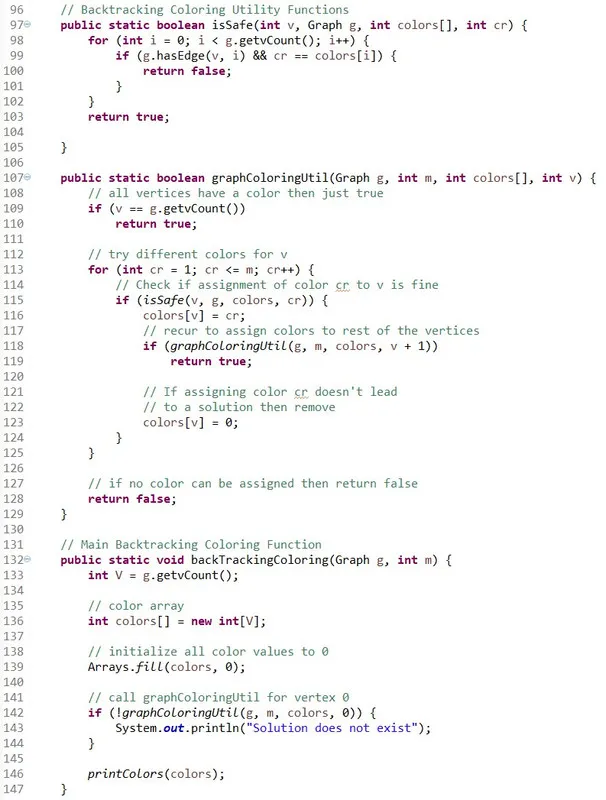[Eclipse Screenshot]

### Code Explanation:

We first have to define two utility/help functions:

• isSafe() -> which checks if the current color assignment is "safe" to set or not, by checking if the colors of the adjacent vertices to 'v' have the color 'cr' that we want to assign to  'v'.
• graphColoringUtil() -> this is the "main" algorithm procedure, where we try different colors for v, by checking if the current assignment is "safe" with isSafe().

The main algorithm function is just initializing the colors[] array, which has the same usage as with the Greedy algorithm, and then calliong the graphColoringUtil() function for vertex 0. When the algorithm is successful it then prints out the solution, else it tells us that no solution exists.

## Testing out the Algorithms

To test out both algorithms I define the TestGraphs class, that also contains these algorithms: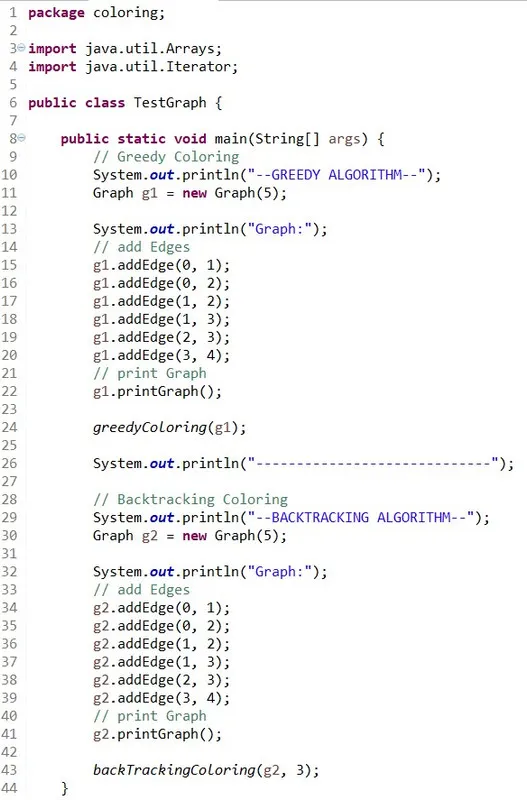[Eclipse Screenshot]

We simply define the same exact Graph twice and run both Algorithms on it...

This gives us the following Console output:As you can see that both algorithms gave the same solution. We can see that the greedy algorithm starts at color 0, whilst the Backtracking algorithm starts at color 1. These can be easily seen if you check the Code of these functions/algorithms.

Also, note that we defined a maximum number of colors of '3' for the second algorithm, which is also the chromatic number of this Graph, as running it with '2' tells us that no solution exists! You can try it our for yourself to also see how it runs! Don't forget that the Code is specified to be contained in a "coloring" package, which you can create to stay organized in the Java IDE. You can also simply remove the first line of both files.

## Final words | Next up on the project

This is actually it for today's post! I hope that you enjoyed it and learned something out of it! For now, the two problems and algorithms that solve them, that I want to implement are:

• The Travelling Salesman Problem
• The Vertex Cover Problem

If you have any suggestion, feel free to shout out your idea!

## GitHub Account:

https://github.com/drifter1Keep on drifting!

H2
H3
H4
3 columns
2 columns
1 column